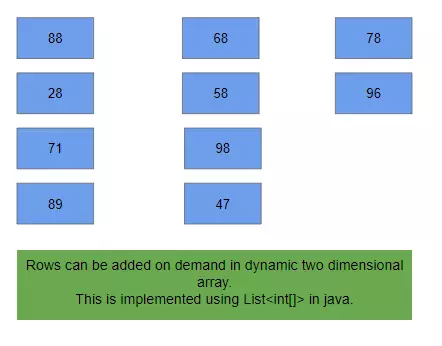In the majority of cases once a two dimensional array is created then the number of rows and columns remains the same, but sometimes you want it to be dynamic. Dynamic two dimensional array in Java is used to have varying numbers of rows where user can add or remove rows on demand. It is implemented using a combination of List and int[]. As the list can grow and shrink hence the 2d array becomes dynamic.## Program to demonstrate dynamic two dimensional array

We will look at:

1. How to create a dynamic 2d array using a List<int[]>.
2. How to add rows to it.
3. Print the rows, and then add some more rows.
```import java.util.ArrayList;
import java.util.Arrays;
import java.util.List;

public class DynamicTwoDimensionalArray {
public static void main(String[] args) {
List<int[]> values = new ArrayList<int[]>();
values.add(new int[] { 88, 66, 78 });
values.add(new int[] { 28, 58, 96 });
values.add(new int[] { 71, 98 });
// print data of each row
for (int[] eachRow : values) {
System.out.println(Arrays.toString(eachRow));
}
values.add(new int[] { 89, 47 });
// print data of each row
for (int[] eachRow : values) {
System.out.println(Arrays.toString(eachRow));
}
}
}
```

Output:

```[88, 66, 78]
[28, 58, 96]
[71, 98]